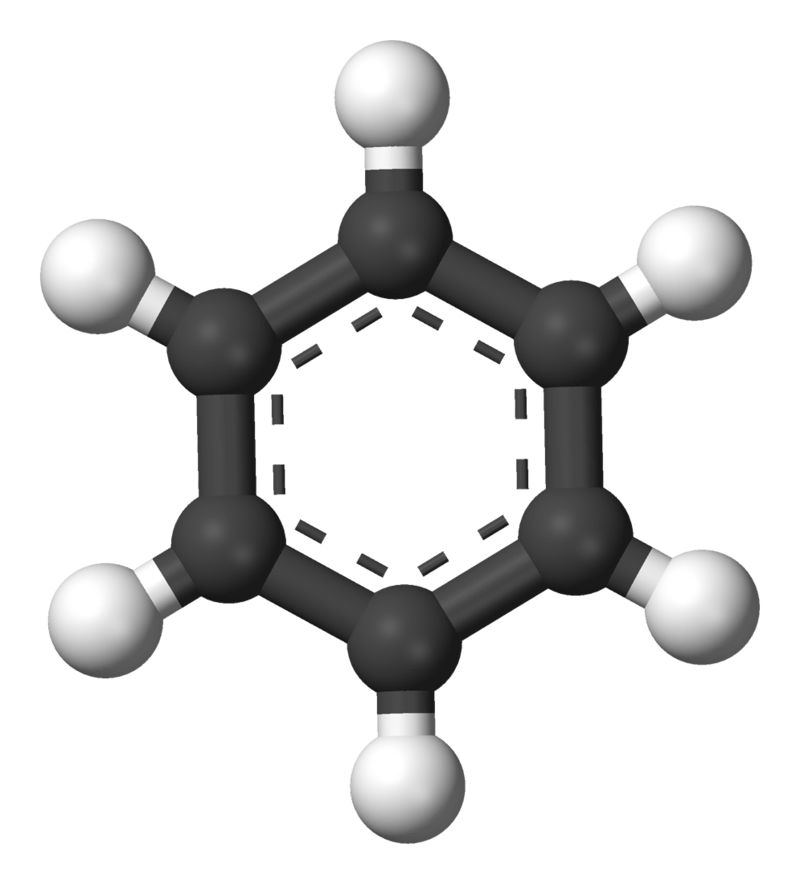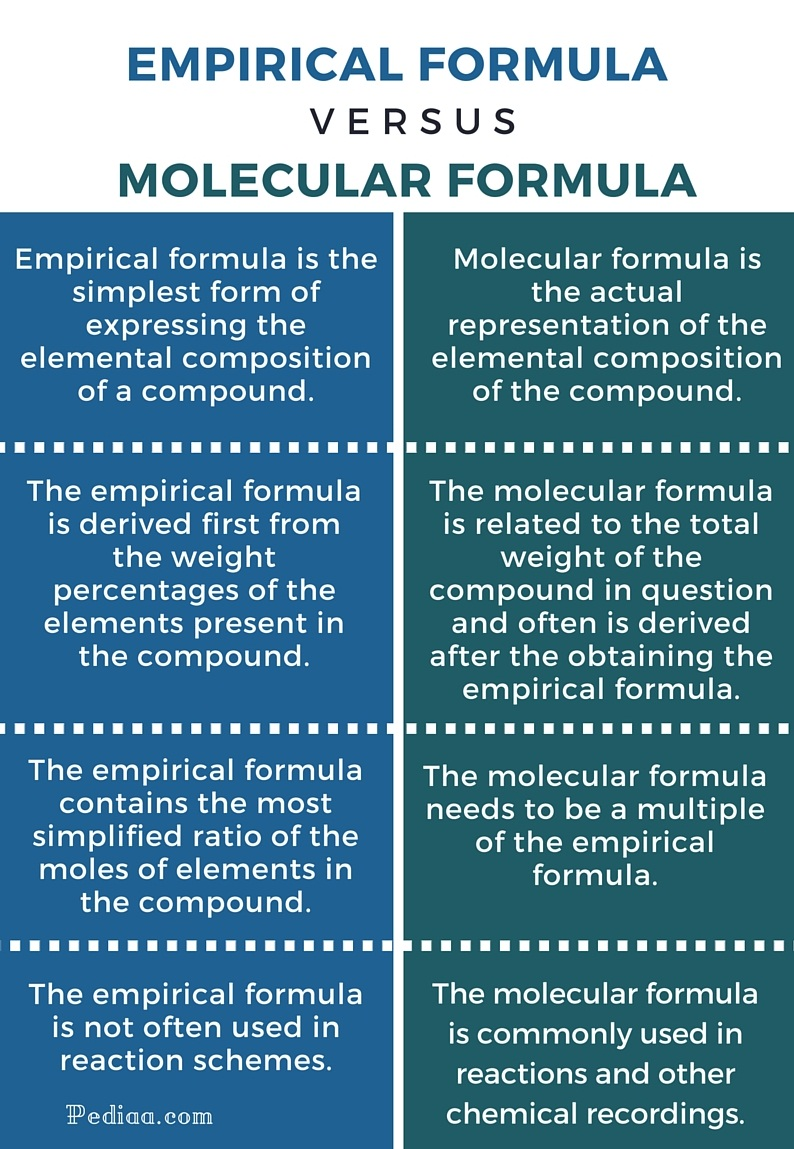# Difference Between Empirical and Molecular Formula

## Main Difference – Empirical vs Molecular Formula

In chemical literature, the term ‘formula’ refers to the written composition of a compound. There are different ways in recording the composition of a compound and they carry different meanings. The empirical form of recording and the molecular form of recording are two such ways. The word ‘empirical’ means that ‘the results are obtained experimentally’. However, when applied in the context of a formula, an empirical formula is the simplest type of formula used to express the composition of a compound whereas the molecular formula is the exact representation of the actual compound. This is the main difference between empirical and molecular formula.

## What is an Empirical Formula

As mentioned above, this is the simplest form of a formula. It only gives information of the ratio in which each element is present in the formula against the others. Usually, the empirical formula is taken down when the composition of elements in the compound is presented as weight percentages. Therefore, in order to come up with the empirical formula, what needs to be done is to assume that the total weight of the compound is 100g. This way, each percentage amount can be directly translated as the elemental weight in the compound. And a comparison of the weights of the elements in grams can be obtained.

However, the formula represents the elements according to their stoichiometric ratios. This means that the weights need to be converted into moles. The conversion can be achieved by simply dividing each weight in grams by the respective molar weight (atomic mass unit) of the element. It would result with the number of moles present for each element. Then these numbers can be compared as ratios and further simplified till the simplest ratio combination. What is left with would be the empirical formula for the compound in question.

## What is a Molecular Formula

The molecular formula gives you the exact elemental composition of a compound. This can be the simplest form, which is the empirical formula, or a simple multiple of it. The molecular formula will always be a multiple of the empirical formula. If not, it would be considered an error.

When finding the molecular formula of a compound, the total weight of the compound is necessary (assumptions as in the case for the empirical formula are not valid here). And afterwards, the total weight obtained needs to be compared with an elemental breakdown in the number of moles as given in the empirical formula and the molar weights of each element. Therefore, each number of moles of an element is multiplied by its respective molar weight and they are added together. Next, this entire expression is multiplied by a certain ‘factor’ and is equalled to the total weight measured. By this, it is possible to find the value of the ‘factor’ which was incorporated in the expression to the nearest whole number. Then this found value is used to multiply the number of moles of each element in the empirical formula. The result would be the molecular formula of the compound.Empirical formula of Benzene is CH. Molecular formula of Benzene is C6H6.

## Difference Between Empirical and Molecular Formula

### Definition

Empirical formula is the simplest form of expressing the elemental composition of a compound.

Molecular formula is the actual representation of the elemental composition of the compound.

### Process of Derivation

The empirical formula is derived first from the weight percentages of the elements present in the compound.

The molecular formula is related to the total weight of the compound in question and often is derived after the obtaining the empirical formula.

### Relationship Between Empirical and Molecular Formula

The empirical formula contains the most simplified ratio of the moles of elements in the compound.

The molecular formula needs to be a multiple of the empirical formula.

### Usage

The empirical formula is not often used in reaction schemes.

The molecular formula is commonly used in reactions and other chemical recordings.

### Nomenclature

The empirical formula is not used for naming purposes as an empirical formula can result in any number of molecular formulas.

A compound’s molecular formula is used to name it.Image Courtesy:

“Benzene-aromatic-3D-balls” by Benjah-bmm27 – Own work. (Public Domain) via Commons